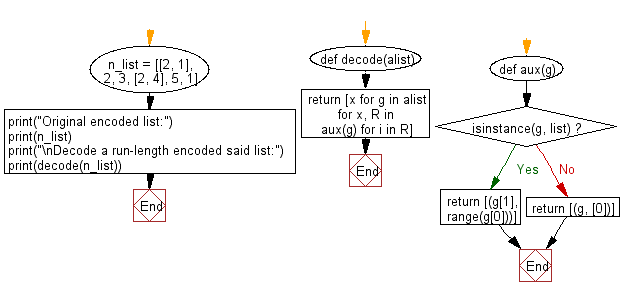﻿ Python: Decode a run-length encoded given list - w3resource# Python: Decode a run-length encoded given list

## Python List: Exercise - 77 with Solution

Run-length encoding (RLE) is a form of lossless data compression in which runs of data (sequences in which the same data value occurs in many consecutive data elements) are stored as a single data value and count, rather than as the original run.

Write a Python program to decode a run-length encoded given list.

Sample Solution:

Python Code:

``````def decode(alist):
def aux(g):
if isinstance(g, list):
return [(g, range(g))]
else:
return [(g, )]
return [x for g in alist for x, R in aux(g) for i in R]
n_list = [[2, 1], 2, 3, [2, 4], 5, 1]
print("Original encoded list:")
print(n_list)
print("\nDecode a run-length encoded said list:")
print(decode(n_list))
```
```

Sample Output:

```Original encoded list:
[[2, 1], 2, 3, [2, 4], 5, 1]

Decode a run-length encoded said list:
[1, 1, 2, 3, 4, 4, 5, 1]
```

Flowchart:## Visualize Python code execution:

The following tool visualize what the computer is doing step-by-step as it executes the said program:

Python Code Editor:

Have another way to solve this solution? Contribute your code (and comments) through Disqus.

What is the difficulty level of this exercise?

Test your Python skills with w3resource's quiz

﻿

## Python: Tips of the Day

Floor Division:

When we speak of division we normally mean (/) float division operator, this will give a precise result in float format with decimals.

For a rounded integer result there is (//) floor division operator in Python. Floor division will only give integer results that are round numbers.

```print(1000 // 300)
print(1000 / 300)```

Output:

```3
3.3333333333333335```